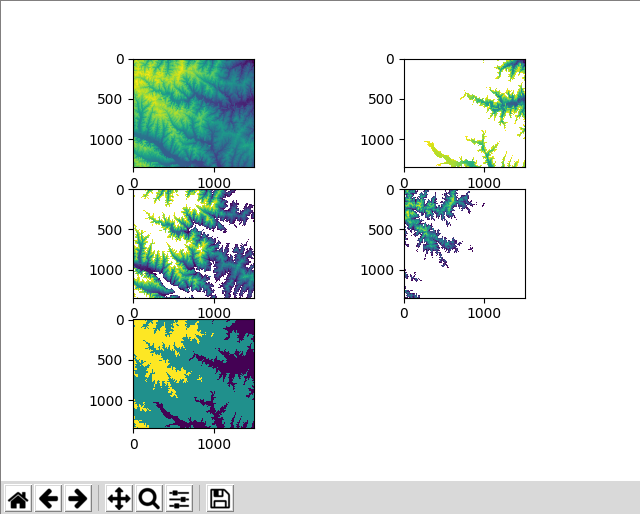# How to classify a GeoTIFF file using NumPy

Institute for Environmental and Spatial Analysis...University of North Georgia

## 1   Introduction

Try these exercises first:

In this cell extraction exercise, we extracted cell values as 1-dimensional arrays, but they are not maps and lost location information. Now, let’s try to classify a GeoTIFF file into multiple classes and retain cell locations as 2-dimensional arrays. Use elevation.tif.## 2   Python code

``````from osgeo import gdal
import numpy as np
import matplotlib.pyplot as plt

ds = gdal.Open(filename)
band = ds.GetRasterBand(1)
del ds, band
return arr

elev_mean = elev_arr.mean()
elev_std = elev_arr.std()

# use np.where() to extract 2-dimensional subsets of elev_arr
low_arr = np.where(elev_arr < elev_mean-elev_std, elev_arr, np.nan)
middle_arr = np.where((elev_arr >= elev_mean-elev_std) &
(elev_arr <= elev_mean+elev_std), elev_arr, np.nan)
high_arr = np.where(elev_arr > elev_mean+elev_std, elev_arr, np.nan)

class_arr = np.where(elev_arr < elev_mean-elev_std, 1,
np.where(elev_arr <= elev_mean+elev_std, 2, 3))

plt.subplot(321)
plt.imshow(elev_arr)

plt.subplot(322)
# low_arr is a 2-dimensional array, so we need to use plt.imshow() to plot it
plt.imshow(low_arr)

plt.subplot(323)
plt.imshow(middle_arr)

plt.subplot(324)
plt.imshow(high_arr)

plt.subplot(325)
plt.imshow(class_arr)

plt.show()``````

## 3   Explanation

Import necessary modules and define the `read_geotiff()` function.

``````from osgeo import gdal
import numpy as np
import matplotlib.pyplot as plt

ds = gdal.Open(filename)
band = ds.GetRasterBand(1)
del ds, band
return arr``````

Read data as a 2-dimensional NumPy array and caculate its statistics.

``````elev_arr = read_geotiff("elevation.tif")
elev_mean = elev_arr.mean()
elev_std = elev_arr.std()``````

We use the `np.where()` function to extract subsets of the 2-dimensional array. It takes three arguments including a boolean array, a true array or value, and a false array or value. Its output has the same shape as that of the first boolean array argument. The second and third arguments should be either of the same shape as the first argument or a single value. For each element in the boolean array, if its value is `True`, the value in the true array at the same location as in the first boolean array or the true value is returned to that location in the 2-dimensional output array. The same thing happens for `False` values in the boolean array.

In `low_arr`, we just want to store low elevation cells whose value is less than the mean minus standard deviation, and nothing else for the other cells. We can use `np.nan` to represent NoData cells. Here, `nan` means Not-a-Number. Repeat the same syntax for `middle_arr` and `high_arr`.

``````# use np.where() to extract 2-dimensional subsets of elev_arr
low_arr = np.where(elev_arr < elev_mean-elev_std, elev_arr, np.nan)
middle_arr = np.where((elev_arr >= elev_mean-elev_std) &
(elev_arr <= elev_mean+elev_std), elev_arr, np.nan)
high_arr = np.where(elev_arr > elev_mean+elev_std, elev_arr, np.nan)``````

This time, instead of extracting 2-dimensional cells for low, middle, and high bands, let’s classify them as 1, 2, and 3, respectively, in one array. We still use `np.where()`, but we use it in a nested manner. In short, we use this syntax: `np.where(condition_1, value_1, np.where(condition_2, value_2, value_3))`. If `condition_1` is true, `value_1` is used. Otherwise, `np.where(condition_2, value_2, value_3)` is used. The false case for `condition_1` is again a conditional array. If `condition_2` is true, `value_2` is used. Otherwise, `value_3` is used. Logically, it’s similar to this `if-elif-else` construct.

``````if condition_1 is True:
return value_1
elif condition_2 is True:
return value_2
else:
return value_3``````

However, the construct above is only for single value comparisons and we still need to use `np.where()` to perform cell-by-cell comparisons in a 2-dimensional array.

First, extract low elevation cells using `elev_arr < elev_mean-elev_std`. If this condition is true, use `1`. Otherwise, use `np.where(elev_arr <= elev_mean+elev_std, 2, 3)`. In the otherwise case, we still need to evaluate this truthiness using the second `np.where()`. If a cell in `elev_arr` is not less than the mean minus standard deviation (`elev_arr < elev_mean-elev_std`), but is less than or equal to the mean plus standard deviation (`elev_arr <= elev_mean+elev_std`), use 2. Otherwise (`elev_arr` is not less than `elev_mean-elev_std` and not less than or equal to `elev_mean+elev_std`), use 3. The last case is actually for elevation cells whose value is greater than the mean plus standard deviation.

``````class_arr = np.where(elev_arr < elev_mean-elev_std, 1,
np.where(elev_arr <= elev_mean+elev_std, 2, 3))``````

The `low_arr`, `middle_arr`, `high_arr`, and `class_arr` arrays are all 2-dimensional and can be plotted using `plt.imshow()`.

``````plt.subplot(321)
plt.imshow(elev_arr)

plt.subplot(322)
# low_arr is a 2-dimensional array, so we need to use plt.imshow() to plot it
plt.imshow(low_arr)

plt.subplot(323)
plt.imshow(middle_arr)

plt.subplot(324)
plt.imshow(high_arr)

plt.subplot(325)
plt.imshow(class_arr)

plt.show()``````